# Formula Widgets

The Custom Formula widget provides a way for you to customize your dashboard metrics way beyond the default values provided in other widget types. This widget type can perform mathematical calculations between other widget types such as Lead Acquisition, Engagement, Conversion, and Retention widgets.

For example, if you’d like to find the sum of the values of two separate widgets on the dashboard, you can use a Custom Formula widget to run that type of calculation.

## Creating a Formula Widget

You can add formula widgets to any dashboard in your SegMetrics account.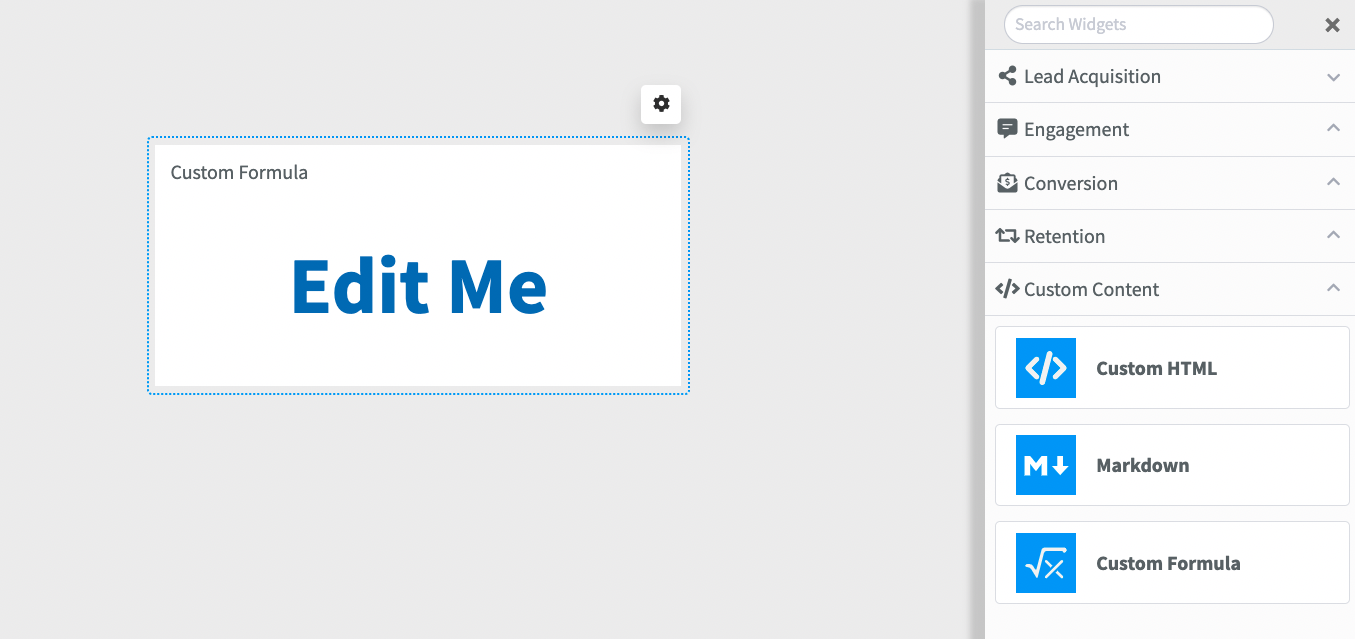Once you’ve opened up a dashboard:

2. Click Custom Content
3. Drag a new Custom Formula widget onto the dashboard
4. Double click the widget to pull up the editor

## Editing Custom Formula Widgets

As mentioned before, formula widgets can perform mathematical calculations. To perform a calculation, enter a mathematical formula into the widget editor: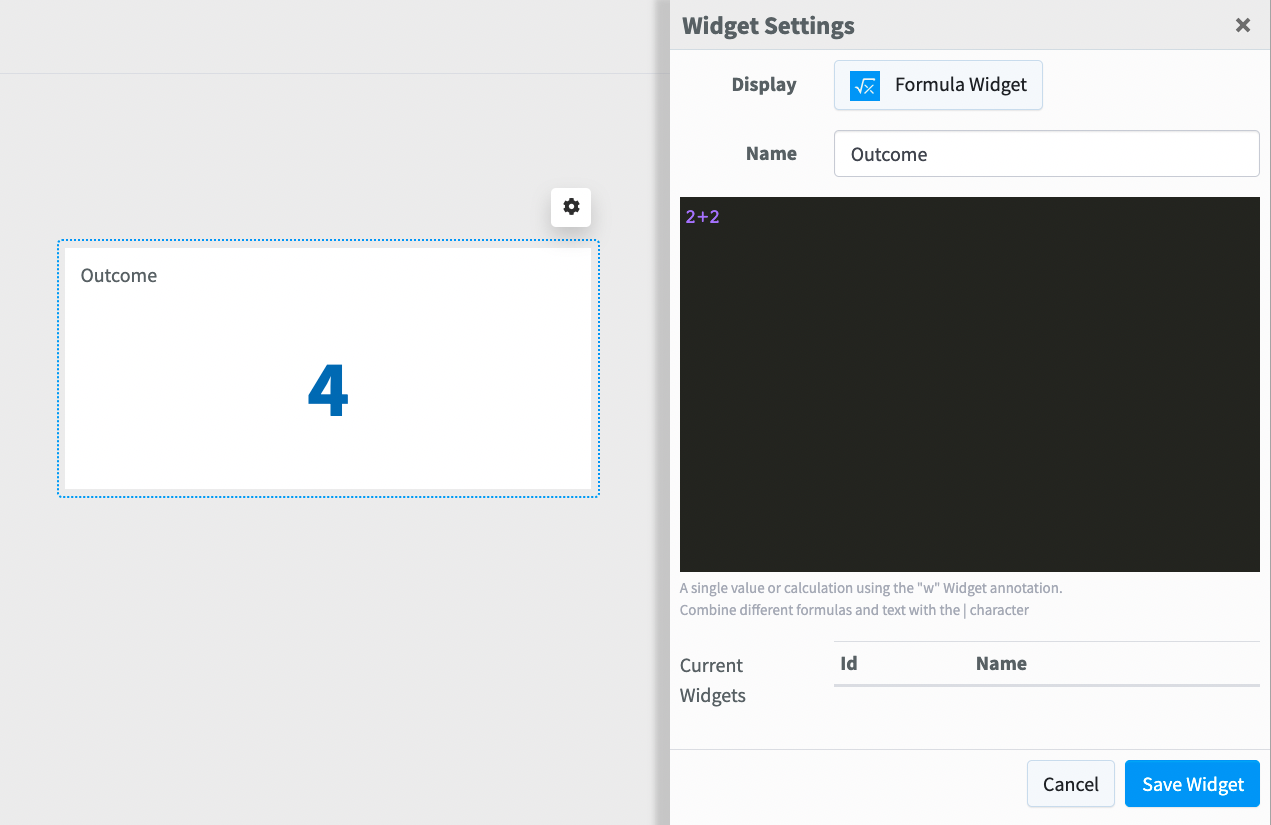If you have other widget types already created on your dashboard, you’ll notice a list of widget IDs and Names. Those widget IDs contain the data about the widget’s metrics. Those widget ID’s can then be used within your calculation: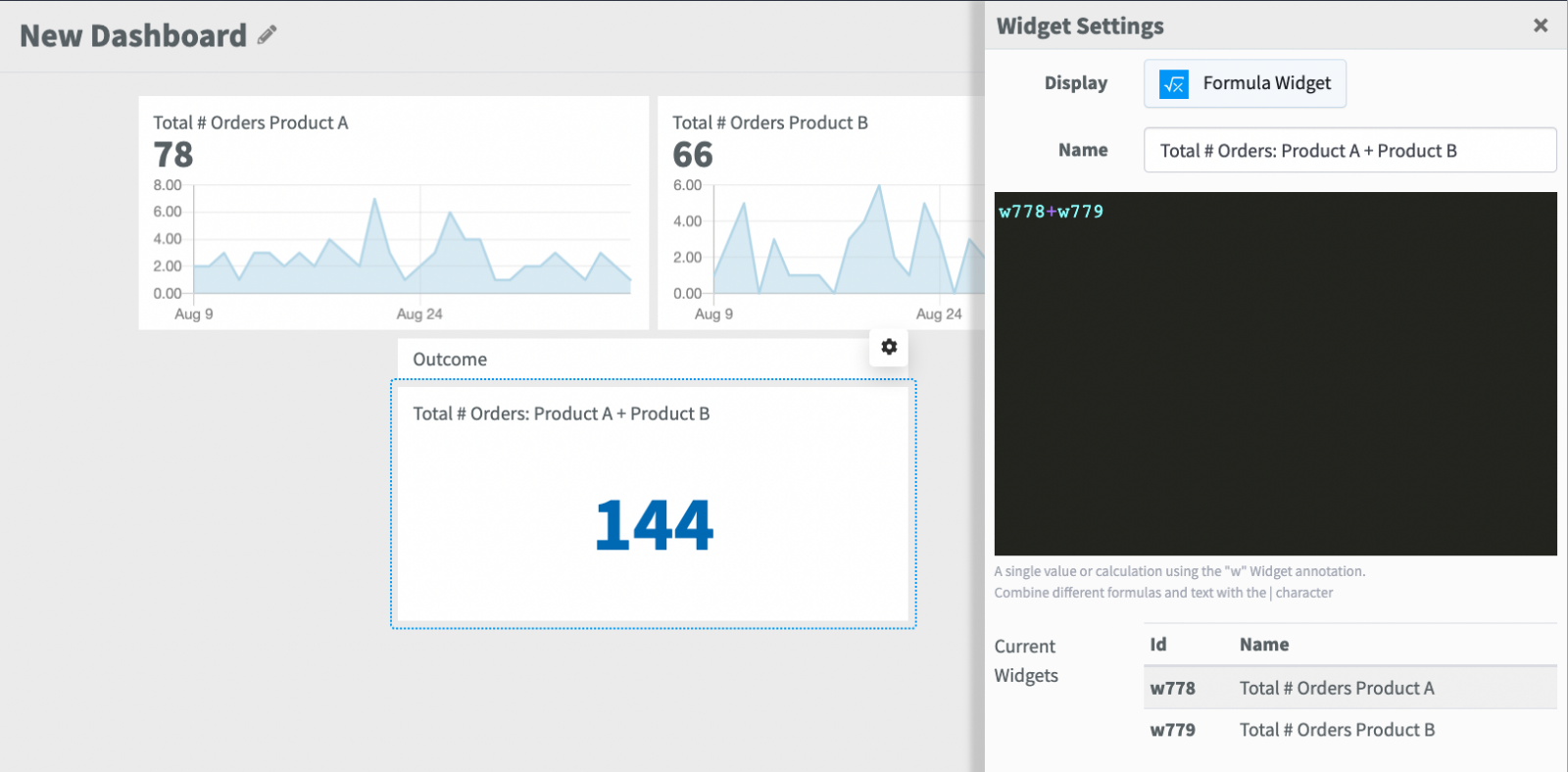It’s also possible to run date based calculations in formula widgets. SegMetrics is integrated with the Moment.js open source library. In fact, you can utilize any moment.js variable that is available in the library. Here is some additional documentation.

To pull the current date, use the following formula: DATE()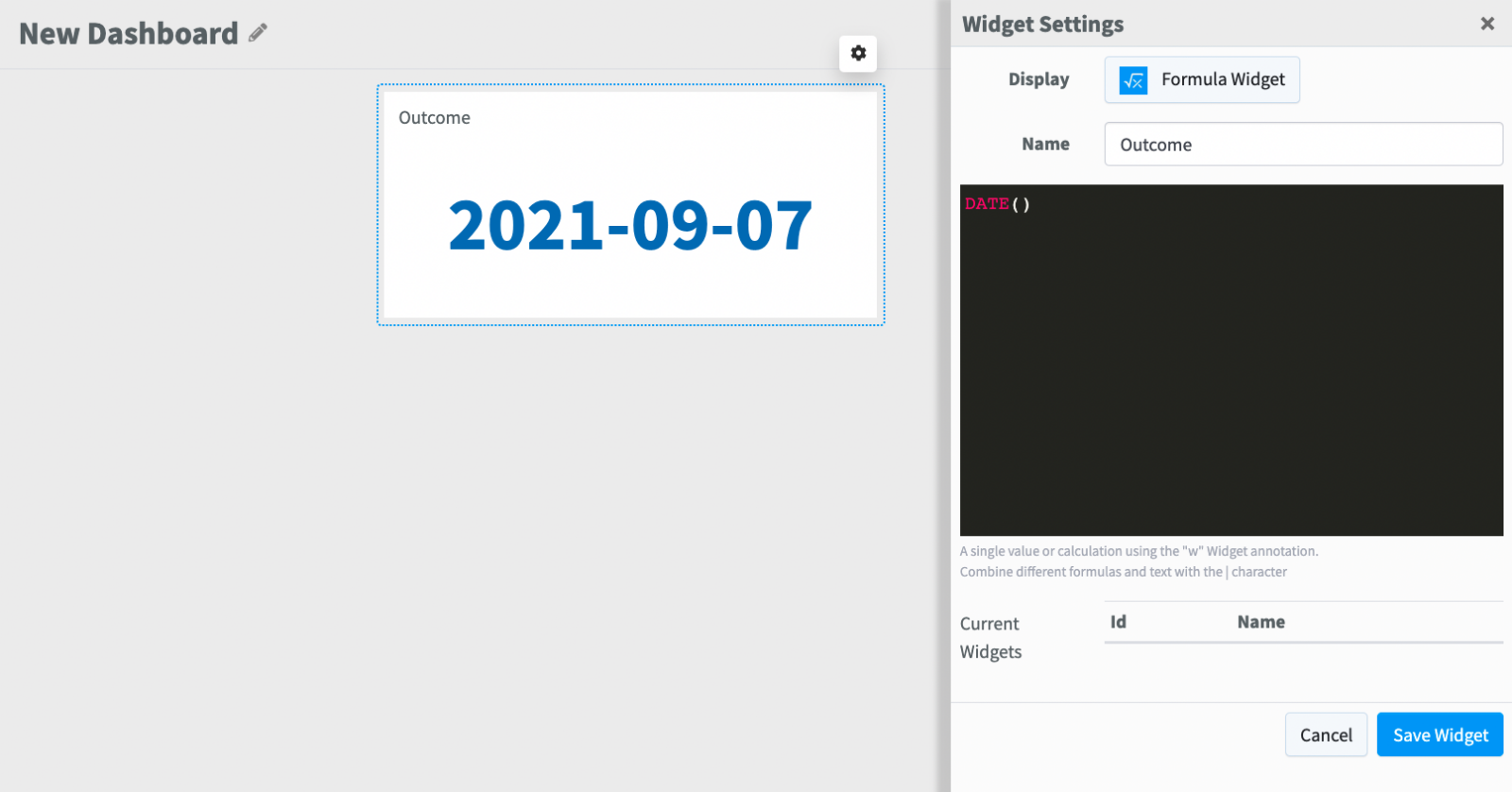As you’ll see, the default date format is: YYYY-MM-DD. To format the date in a different way, insert the preferred format into the parentheses: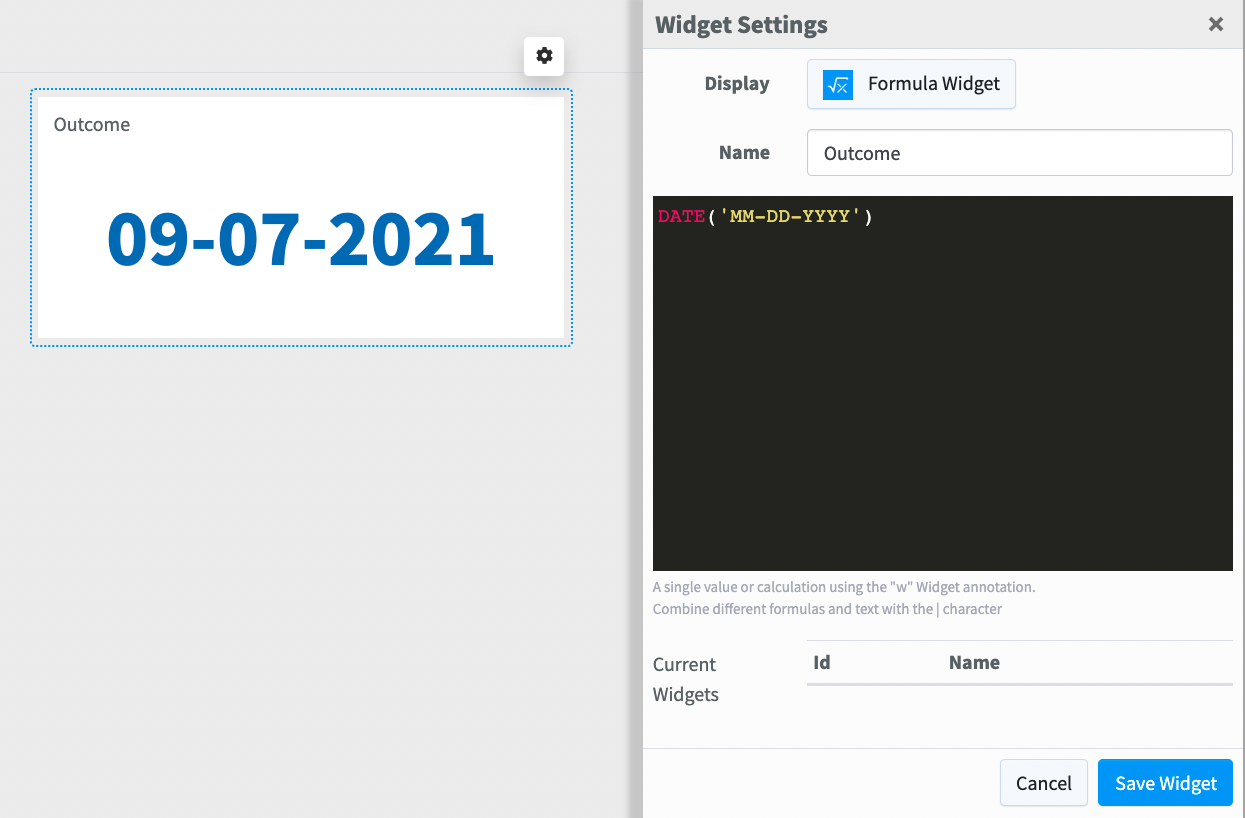Now the date is formatted as MM-DD-YYYY.

The ability to run date based calculations allows you to create forecasts of important metrics such as revenue and new lead growth.We’ve put together a list of the most commonly used formulas that can be used in formula widgets.

## Differences from standard widgets

• Formula widgets always have a blue bar on the right side to denote them as being calculated• You may double-click a Formula widget to edit the formula in-place:## Available Functions

You can use the following functions and formulas to modify your values.
In addition, all Formula widgets support standard math functions (+, -, *, /, ^)

Formula Description
| (pipe character) Concatenate different formulas and text together. Useful for adding text before or after a value
w Use w (followed by a number) to reference the value of an existing widget.
n([number], [decimal]) Format a number, rounding to a number of decimal points
PERCENT([number], [decimal]) Format a decimal as a percentage, rounding to a number of decimal points, and add a percentage sign at the end
DIFF([original value], [new value], [decimal]) Calculate the percentage is change between the original value and the new value, rounding to a number of decimal points, and add a percentage sign at the end
DATE([format], [date]) Format an absolute (2021-02-02) or relative (-2 days) date in the defined format. Format defaults to 'YYYY-MM-DD'
abs(x) Calculate the absolute value of a number.
ceil(x) Round a value up
cube(x) Compute the cube of a value,  `x * x * x`.
exp(x) Calculate the exponent of a value.
floor(x) Round a value down
round(x [, n]) Round a value towards the nearest integer.
sqrt(x) Calculate the square root of a value.
mad(a, b, c, …) Compute the median absolute deviation of a matrix or a list with values.
max(a, b, c, …) Compute the maximum value of a matrix or a list with values.
mean(a, b, c, …) Compute the mean value of matrix or a list with values.
median(a, b, c, …) Compute the median of a matrix or a list with values.
min(a, b, c, …) Compute the minimum value of a matrix or a list of values.
std(a, b, c, …) Compute the standard deviation of a matrix or a list with values.
sum(a, b, c, …) Compute the sum of a matrix or a list with values.
variance(a, b, c, …) Compute the variance of a matrix or a list with values.

## Common Examples

Formula Example Description
n(( LEADS / TODAY_DATE ) * DAYS_IN_MONTH, 0)
Forecast lead generation for the month
n( AD_SPEND * 108.56) | "円" Convert Ad Spend from Dollars to Yen